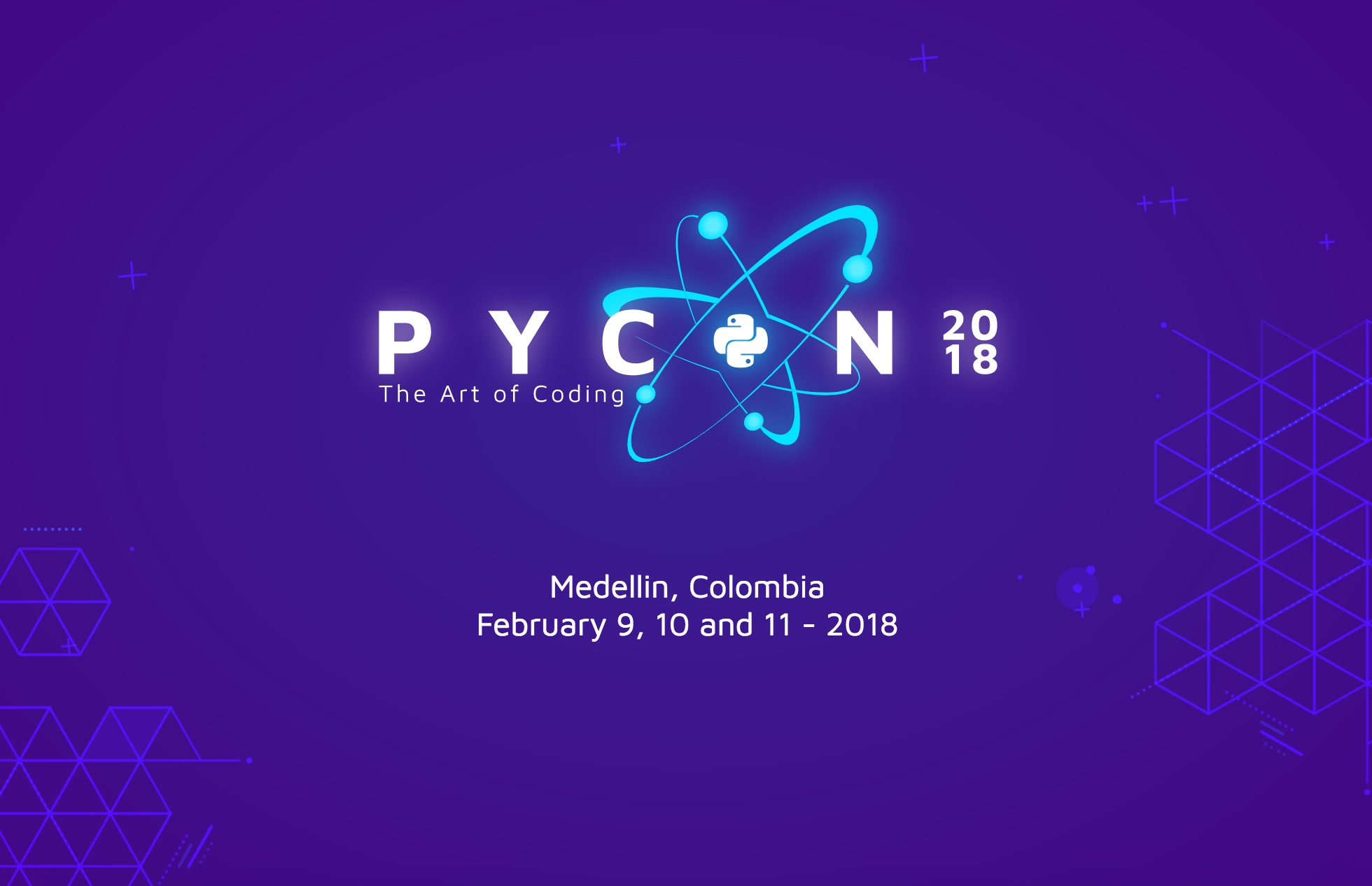# Talk

## Painting Chaos with Python (Spanish)

Location:
Block 38 - Room 110
Date and time:
Friday 09, 11:40
Authors:
Isabel Cristina Ruiz Buriticá (Colombia)
Slides:
painting-chaos-with-python.pdf
Summary:

We will talk about Fractal, its applications, and how computers were essential to be able to represent these complex and striking mathematical forms. The Matplotlib, Numpy and Turtle graphics libraries of Python will be used to graph the main fractals.

Description:

If you want to start building computational designs with patterns beyond the simple `ellipse()`, `rect()` and `line()` forms, it's time to learn about concepts and techniques to simulate the geometry of nature: fractals.

We will review two ways of generating fractals, first, a function that is recursively called and that will always produce the same result in each iteration, applies to classical fractals such as the Cantor set, the Sierpinski triangle, binary trees, etc., and second, combine the recursive algorithms with randomness and generate a different and more realistic result in each iteration, for example, we could use a random color, line thickness, orientation, etc. It applies to complex fractals such as the Koch curves, the Mandelbrot set, and the julia set. The recursive fractal tree is a good example of a scenario in which adding a bit of randomness can make the element look more natural.